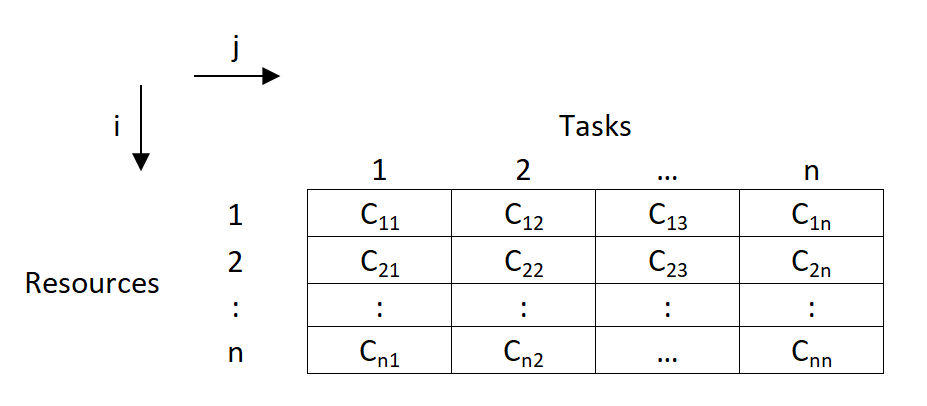## What is Assignment Model?

→ Assignment model is a special application of Linear Programming Problem (LPP), in which the main objective is to assign the work or task to a group of individuals such that;

i) There is only one assignment.

ii) All the assignments should be done in such a way that the overall cost is minimized (or profit is maximized, incase of maximization).

→ In assignment problem, the cost of performing each task by each individual is known.
→ It is desired to find out the best assignments, such that overall cost of assigning the work is minimized.

#### For example:

• Suppose there are 'n' tasks, which are required to be performed using 'n' resources.

• The cost of performing each task by each resource is also known (shown in cells of matrix)• In the above asignment problem, we have to provide assignments such that there is one to one assignments and the overall cost is minimized.

## How Assignment Problem is related to LPP? OR Write mathematical formulation of Assignment Model.

→ Assignment Model is a special application of Linear Programming (LP).

→ The mathematical formulation for Assignment Model is given below:→ Let, $\text {C}_{ij}$ denotes the cost of resources 'i' to the task 'j'; such that

\begin{aligned} x_{ij} &= 1 \ ; \text{if} \ \ i^{th} \text{resource is original to } j^{th} \text{task}\\ x_{ij} &= 0 \ ; \text{if} \ \ i^{th} \text{resource is not original to } j^{th} \text{task} \end{aligned}

→ Now assignment problems are of the Minimization type. So, our objective function is to minimize the overall cost.

$\therefore \text{Minimize Z}= \displaystyle\sum_{j=1}^n \ \sdot \ \displaystyle\sum_{i=1}^n \ C_{ij} \ \sdot \ x_{ij}$

→ Subjected to constraint;

(i) For all $j^{th}$ task, only one $i^{th}$ resource is possible:

$\therefore \displaystyle\sum_{j=1}^n \ x_{ij} = 1 \quad \text{(i=1,2,3, ...,n)}$

(ii) For all $i^{th}$ resource, there is only one $j^{th}$ task possible;

$\therefore \displaystyle\sum_{i=1}^n \ x_{ij} = 1 \quad \text{(j=1,2,3, ...,n)}$

(iii) $x_{ij}$ is '0' or '1'.

## Types of Assignment Problem:

#### (i) Balanced Assignment Problem

• It consist of a suqare matrix (n x n).
• Number of rows = Number of columns

#### (ii) Unbalanced Assignment Problem

• It consist of a Non-square matrix.
• Number of rows $\not=$ Number of columns

## Methods to solve Assignment Model:

#### (i) Integer Programming Method:

• In assignment problem, either allocation is done to the cell or not.

• So this can be formulated using 0 or 1 integer.

• While using this method, we will have n x n decision varables, and n+n equalities.

• So even for 4 x 4 matrix problem, it will have 16 decision variables and 8 equalities.

• So this method becomes very lengthy and difficult to solve.

#### (ii) Transportation Methods:

• As assignment problem is a special case of transportation problem, it can also be solved using transportation methods.

• In transportation methods (NWCM, LCM & VAM), the total number of allocations will be (m+n-1) and the solution is known as non-degenerated. (For eg: for 3 x 3 matrix, there will be 3+3-1 = 5 allocations)

• But, here in assignment problems, the matrix is a square matrix (m=n).

• So total allocations should be (n+n-1), i.e. for 3 x 3 matrix, it should be (3+3-1) = 5

• But, we know that in 3 x 3 assignment problem, maximum possible possible assignments are 3 only.

• So, if are we will use transportation methoods, then the solution will be degenerated as it does not satisfy the condition of (m+n-1) allocations.

• So, the method becomes lengthy and time consuming.

#### (iii) Enumeration Method:

• It is a simple trail and error type method.

• Consider a 3 x 3 assignment problem. Here the assignments are done randomly and the total cost is found out.

• For 3 x 3 matrix, the total possible trails are 3!
So total 3! = 3 x 2 x 1 = 6 trails are possible.

• The assignments which gives minimum cost is selected as optimal solution.

• But, such trail and error becomes very difficult and lengthy.

• If there are more number of rows and columns,
( For eg: For 6 x 6 matrix, there will be 6! trails. So 6! = 6 x 5 x 4 x 3 x 2 x 1 = 720 trails possible)
then such methods can't be applied for solving assignments problems.

#### (iv) Hungarian Method:

• It was developed by two mathematicians of Hungary. So, it is known as Hungarian Method.

• It is also know as Reduced matrix method or Flood's technique.

• There are two main conditions for applying Hungarian Method:

(1) Square Matrix (n x n).
(2) Problem should be of minimization type.